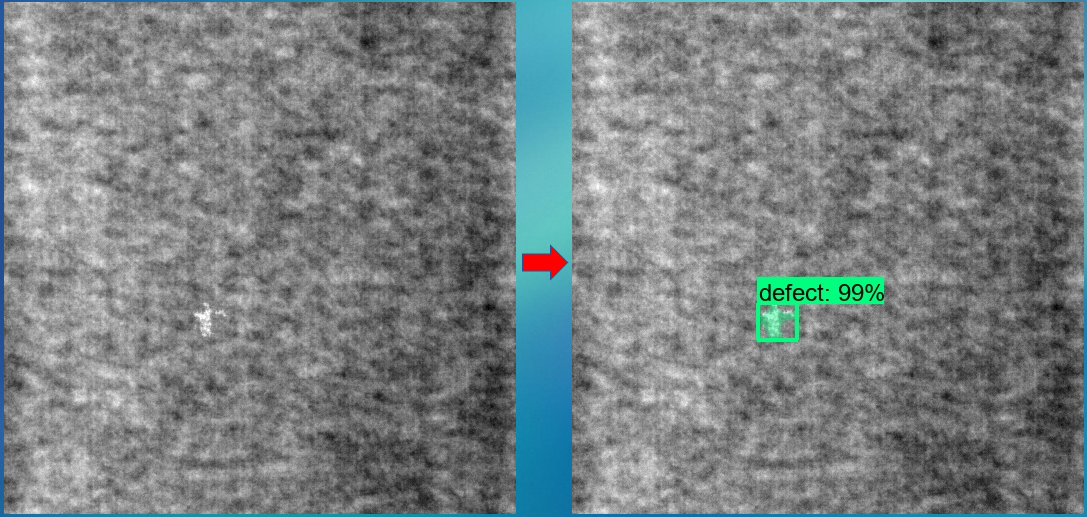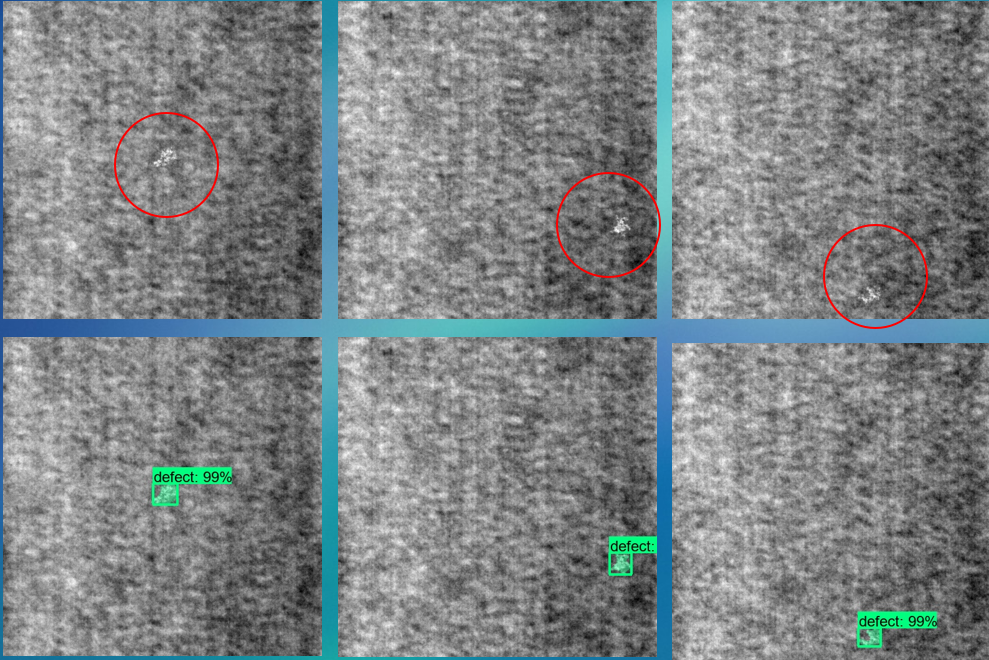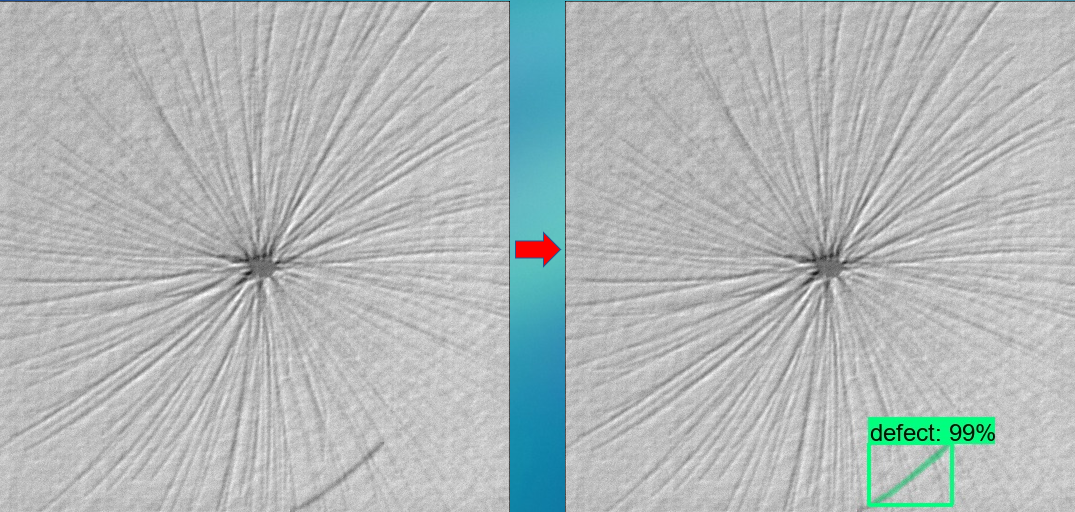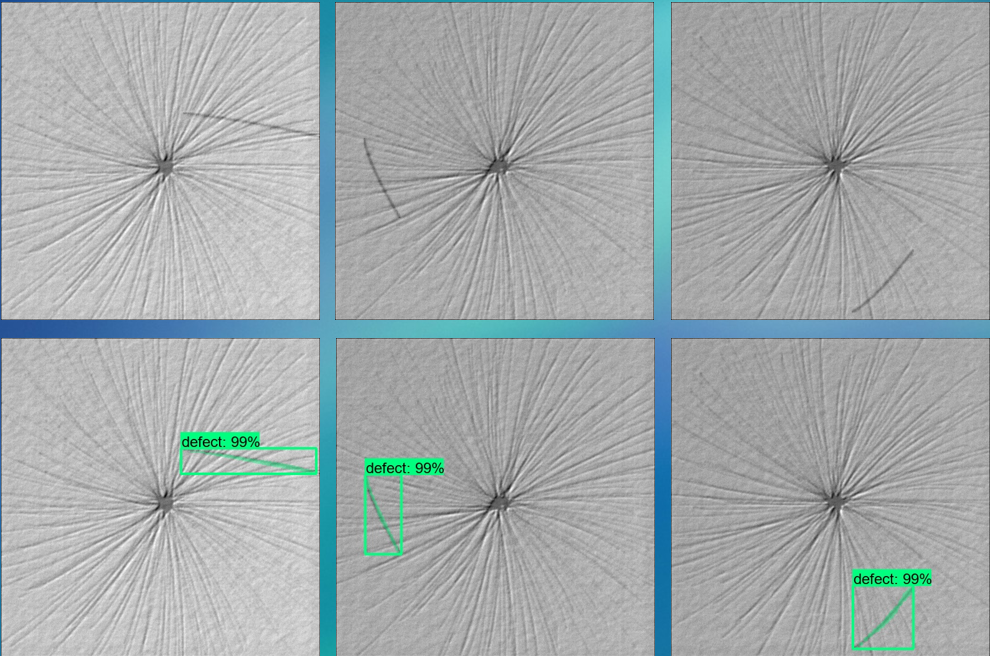• 硅片缺陷检测算法,包括程序代码和数据集，检测精度高．
•研究论文
•研究论文
• 基于机器视觉的 PCB 缺陷检测算法研究，博硕论文，基于机器视觉的 PCB 缺陷检测算法研究
• 二维码印刷缺陷检测算法研究，董成松，周亚建，针对二维码印刷过程中的出现的缺陷问题，提出一种二维码印刷缺陷检测算法，并给出了该算法的工作流程以及用到的数学模型，该算法
•研究论文
• 基于Gabor滤波器和低秩分解的织物缺陷检测算法研究论文
• 设计了一种基于图像匹配技术的缺陷检测算法，实现了字符缺陷的自动化检测．介绍了检测装置的总体框架和检测算法，算法的核心是提出的改进的基于形状模板的图像匹配算法，利用加权归一化向量点积相似度量计算方法，...
• 基于图像数据重排的产品表面缺陷检测算法研究.pdf
• 文章在全面分析软件系统安全性缺陷的基础上，提出一种基于相似特征的软件安全性缺陷检测算法。针对C语言源代码，应用实例推理CBR的技术原理，通过检测算法将源代码的安全特征与已知安全性缺陷的实例特征进行相似匹配...
• Ｃ++语言开发，胶囊缺陷检测程序，里面也包括胶囊图片数据集，检测准确率高．
• 为了提高工业产品质量控制中表面缺陷检测的准确性和可靠性，提出一种基于多元图像分析(MIA)的表面缺陷检测算法．采用合格样本图像和被测图像堆叠构成多元被测图像，利用基于主成分分析(PCA)的多元图像处理方法，获得...
• https://zhuanlan.zhihu.com/p/107436354 深度学习实现缺陷检测算法汇总
https://zhuanlan.zhihu.com/p/107436354
深度学习实现缺陷检测算法汇总


展开全文• 为了满足工业上对织物缺陷检测的实时性要求,提出一种基于S-YOLOV3(Slimming You Only Look Once Version 3)模型的织物实时缺陷检测算法。首先使用K均值聚类算法确定目标先验框,以适应不同尺寸的缺陷;然后预训练YOLOV...
• 数字图像处理作业——隐形眼镜缺陷检测算法 摘 要：本文致力于寻找出一种具有较强鲁棒性的检测隐形眼镜边缘缺陷的方法。本文针对图像中物体几何形状的特殊性，提出了一种基于霍夫变换的缺陷检测算法，并在低噪声图像...
数字图像处理——隐形眼镜缺陷检测算法
摘 要：本文致力于寻找出一种具有较强鲁棒性的检测隐形眼镜边缘缺陷的方法。本文针对图像中物体几何形状的特殊性，提出了一种基于霍夫变换的缺陷检测算法，并在低噪声图像的缺陷检测中取得了不错的效果。在此过程中，本文还分别对残缺图像、大缺陷图像以及含椒盐噪声的图像进行了实验，仅通过改变预处理的一些步骤就可达到相同的检测效果，验证了该算法的鲁棒性。
关键词：霍夫变换；边缘检测；缺陷检测；机器视觉；图像处理
Abstract: This paper aims to find a robust method to detect the edge defects of contact lenses. In this paper, a defect detection algorithm based on Hough transform is proposed according to the particularity of object geometry in the image, and good results are obtained in the defect detection of low noise image. In this process, experiments are carried out on incomplete images, large defect images and images with salt and pepper noise. The same detection effect can be achieved only by changing some steps of preprocessing, which verifies the robustness of the algorithm.
Key Words: Hough transform; edge detection; defect detection; machine vision; image processing
目录数字图像处理——隐形眼镜缺陷检测算法摘要隐形眼镜图像缺陷检测方法理想图像的缺陷检测霍夫圆变换简介残缺图像的缺陷检测含大缺陷图像的缺陷检测含椒盐噪声图像的缺陷检测结论参考文献：附录MATLAB代码：原图处理部分椒盐噪声处理部分其他算子（孔洞填充&霍夫变换）
摘要
随着现代工业的发展，基于机器视觉的缺陷检测方法在工业生产中有着极大的应用空间。然而在实际的缺陷检测过程中，由于硬件设施的局限性与环境的复杂性，不得不考虑各种例如相机畸变、信号传输或者灰尘污染等实际问题带来的干扰。本文主要考虑了三种干扰情况：图像残缺，大缺陷物件，椒盐噪声。这三类情况对算法鲁棒性的要求都相对较高。因此，开发一种满足较强鲁棒性的缺陷检测算法，是亟待解决的关键问题。
隐形眼镜图像缺陷检测方法
理想图像的缺陷检测
图1为一张存在缺陷的隐形眼镜图像，隐形眼镜的右下角存在缺陷。需要将缺陷检测出来，首先应该确定缺陷的定义。
由图像可知，图像中半径与其他边缘点不同的地方即为缺陷。检测问题就可以转化为如何求得隐形眼镜的圆心与半径。本文采用霍夫变换圆检测方法来确定圆心与半径，并取得了不错的实验效果。图1 存在缺陷的隐形眼镜图像
由于霍夫变换输入的是图像的二值化边缘图，由此需要先对图像进行一点预处理，将隐形眼镜的边缘提取出来。对于图1，笔者采用了Prewitt算子提取边缘信息。Prewitt算子是一种一阶微分算子，利用像素点上下左右邻点灰度差，在边缘处达到极值检测边缘，对噪声具有平滑的作用。
对数字图像$f\left(x,y\right)$，有如下公式：
$G\left(i\right)=\left|\left[f\left(i-1,j-1\right)+f\left(i-1,j\right)+f\left(i-1,j+1\right)\right]-\left[f\left(i+1,j-1\right)+f\left(i+1,j\right)+f\left(i+1,j\right)+f\left(i+1,j+1\right)\right]\right| （1）$
$G\left(j\right)=\left|\left[f\left(i-1,j+1\right)+f\left(i,j+1\right)+f\left(i+1,j+1\right)\right]-\left[f\left(i-1,j-1\right)+f\left(i,j-1\right)+f\left(i+1,j-1\right)\right]\right| （2）$
Prewitt算子为：
$P\left(i,j\right)=\max{\left[G\left(i\right),G\left(j\right)\right]}（3）$图2 边缘提取后的二值化图像
二值化后的图像如图2所示。
霍夫圆变换简介
在数字图像处理中，霍夫变换理论上可以检测出图像中任何可用解析几何方程表达的规则图形。霍夫变换在直线检测、圆或椭圆检测中得到了广泛的应用。
对于任意圆形，在笛卡尔坐标系中，方程表示为：
$\left(x-a\right)^2+\left(y-b\right)^2=r^2\ （4）$
其中，圆心的坐标为\left(a,b\right)，半径为r。当半径一定，且图形的边缘坐标已知时，图形边缘上的每一个点\left(x_0,y_0\right)，在由参数a，b构成的平面坐标系中为一个圆。此时，方程可改写为：
$\left(a-x_0\right)^2+\left(b-y_0\right)^2=r_0^2 （5）$
若多点共圆，则这些点由（2）式得到的若干圆交于一点，找出此交点即可确定该圆形。然而在实际处理过程各点可能存在一定偏差，无法形成一个完美的交点，故通过投票等方式找出霍夫空间中亮度最高的区域，从而确定圆心与半径。图3即为找出的霍夫圆与圆心的位置。图3 霍夫圆与圆心位置
找出圆心位置与半径后，通过计算边缘图中每一点与圆心的距离，并设定一个区间。将区间之外的点定义为缺陷：
$\{_{e_{min}
其中, $e_{max}$与$e_{min}$为设定好的边界值，满足上述式子的点$\left(x_0,y_0\right)$为边界，否则为缺陷。最后将缺陷点在图像中标注出来。结果如图4。图4  缺陷检测结果
残缺图像的缺陷检测
若图像存在残缺，或摄像头并不能完整照出图像，如图5即为一张存在缺陷的图像。图5  残缺的隐形眼镜图像
由于霍夫变换采用投票的方式找出霍夫空间中亮度最高的区域，确定圆心与半径。对于残缺程度不大的图像仍然能维持一定的鲁棒性。检测效果如图6，图7所示。图6  残缺图像的霍夫变换图像图7  残缺图像的缺陷检测效果
含大缺陷图像的缺陷检测
由于霍夫变换采用投票的方式找出霍夫空间中亮度最高的区域，确定圆心与半径。对于含有离群点的图像仍然能维持一定的鲁棒性。待检测图像如图8所示。图8  含有大缺陷的隐形眼镜图像
但是，由于缺陷较大，需要对先前确定的（6）式中的$e_{min}$与$e_{max}$值做一些调整。检测效果如图9，图10所示。图9  大缺陷图像的边缘二值化图图10  大缺陷图像的缺陷检测效果
含椒盐噪声图像的缺陷检测
由于实际情况下图片中都会含有一定的噪声，本文也探究了含有椒盐噪声情况下图像的缺陷检测方法。
去除椒盐噪声最常用的算法是中值滤波，中值滤波原理如下：
若一个像素点的值为$9$，该像素点及其$3*3$的临近像素点结构元的值如表1所示，中值滤波后的中心像素点的值为这九个数的中位数。

4
7
8

2
9
6

4
1
5

表1  中值滤波元结构示例

\
\
\

\
5
\

\
\
\

表2  中值滤波结果
滤波结果如图11所示（原图信噪比0.9）：图11  含有椒盐噪声的图像（信噪比0.9）图12  中值滤波后的隐形眼镜图像
滤波后的图像如图12所示，二值化后的效果如图13。从图13可以看出图像中仍然含有一定的噪声，将提取边缘后可以采用对于一些小的噪点可以采用含有阈值的孔洞填充将其去除，同时使用形态学膨胀操作突出边界特征。
带阈值的孔洞填充算法基本思想如公式7所示。
$X_k=\left(X_{k-1}\oplus B\right)\cap A^c,k=1,2...（7）$
首先找出所有孔洞的位置，只需知道洞中的一个点的坐标即可；新建一张全零图，用0表示背景，1表示前景，大小与原图相同；取出一个洞的坐标，对该新图用一个结构元进行膨胀，然后再与原图的反（此时含有孔洞的地方应全为1）求与，即对该点执行公式（7）中的操作；如果检测到一次操作完的结果与操作前相同，则结束迭代，再取出下一个洞的坐标，返回到先前操作，直到所有洞都填充完成；完成填充后，此时新图中为数值为1的位置即为需要填补的孔洞，将新图与原图相或即得到孔洞填充完成的图像。
由于每个被填充的孔洞都属于一个连通域，通过其领域的迭代算出该连通域的面积，再删除面积小于一个阈值的连通域即可。处理后的二值化图像如图14所示。图13  中值滤波后二值化图像图14  孔洞填充、形态学膨胀后的二值化图像
最终检测结果如图15。可以看到检测结果仍能保持准确性。图14  中值滤波后图像缺陷检测效果
通过实验，在信噪更低的情况下，该算法仍然可以较准确的检测出缺陷的位置。图16  信噪比0.8的图像图17  信噪比0.8时检测结果图18  信噪比0.7的图像图19  信噪比0.7时检测结果
在信噪比达到0.5时，该算法检测失效。图20  信噪比0.5图像图21  检测失效
结论
经过本文的探究与验证，验证了基于霍夫变换的缺陷检测方法具有较好的鲁棒性与适应性，可以广泛的运用到对规则几何图形的检测中。同时应当注意到的是，霍夫变换在扫描步长与旋转角度取较小值的情况下，虽然检测精度有所提升，但也存在耗费时间较长的问题。故在该算法的实际使用过程中，需要根据实际需求与效果调整算法中的步长参数。
总而言之，基于霍夫变换的缺陷检测有着很好的适用性与潜力。
参考文献：
 Richard O. Duda and Peter E. Hart. Use of the Hough Transformation to Detect Lines and Curves in Pictures (PDF). Artificial Intelligence Center (SRI International). April 1971.
 张强, 王正林. 精通MATLAB图像处理[M]. 电子工业出版社, 2009.
 高浩军, 杜宇人. 中值滤波在图像处理中的应用[J]. 信息化研究, 2004, 30(008):35-36.
 董继扬, 张军英. _种筒单的椒盐噪声滤波算法[J]. 计算机工程与应用, 2003(20):27-28+65.
 江峰, 杜军威, 眭跃飞, et al. 基于边界和距离的离群点检测[J]. 电子学报, 2010(03):206-211.
	李文斌 , 王长松. 基于边界信息的孔洞填充算法[J]. 计算机工程与设计, 2008, 29(015):3958-3959,3962.
	雷丽珍. 数字图像边缘检测方法的探讨[J]. 测绘通报, 2006, 2006(3):40-42.
附录
MATLAB代码：
原图处理部分
clear all;clc;close all;
Im = imread('ContactLens_broke.png');
figure;imshow(Im),title('原图'); hold on;
Im = rgb2gray(Im);

% for i=1:532;
%     for j=1:937;
%         Im(i,j)=0;
%     end
% end
thresh = graythresh(Im);%OTSU
[H,W]=size(Im);
%Im = imcrop(Im,[60,60,800,800]);
%预处理z
BW = im2bw(Im,thresh);%二值化
BW = imcomplement(BW);
%figure;imshow(BW),title('二值化图'); hold on;
prewittBW=edge(Im,'prewitt');%prewitt边缘算子
%DoubleBW=im2double(BW);
%DoubleBW(prewittBW)=1;% 将边缘显示为白色
DoubleBW=zeros(H,W);
DoubleBW(prewittBW)=1;
DoubleBW = im2bw(DoubleBW,0.1);
% dilate=strel('square',3);
% DoubleBW=imdilate(DoubleBW,dilate);
% erode=strel('square',3);
% DoubleBW=imerode(DoubleBW,erode);

%BW = im2bw(DoubleBW,0.2);

subplot;imshow(DoubleBW),title('二值化图'); hold on;
imwrite(DoubleBW,"./DoubleBW.jpg");
[hough_space,hough_circle,para] = hough_Circle(DoubleBW,1,1,300,450,0.8);
figure;imshow(hough_circle),title('霍夫变换');hold on;
X0 = mean(para(2,:));Y0 = mean(para(1,:));Radius=mean(para(3,:));
plot(X0,Y0,"*");
imwrite(hough_circle,"hough.jpg");
%求损坏区域
Index=find(DoubleBW>0);
vX = floor( ( Index - 1 ) / H ) + 1;
vY = mod( Index - 1, H ) + 1;
vDiff = abs( Radius - sqrt( ( vX - X0 ) .^ 2 + ( vY - Y0 ) .^ 2 ) );
vDefectIdx = find( vDiff >3.2 );%& vDiff < 6 );
vDefectX = vX( vDefectIdx );
vDefectY = vY( vDefectIdx );
figure; imshow( Im ); axis image; colormap( gray ); title( 'Detected defects' );
hold on; plot( vDefectX, vDefectY, 'ro' );


椒盐噪声处理部分
clear all; clc; close all;
Im=imread( 'ContactLens.tif' );
Im=Im(:,:,1);
[H,W]=size(Im);
IMHalf=zeros(H/2,W);
for i=1:H/2
IMHalf(i,:)=Im(i+H/2,:);
end
IMSlice=zeros(H/2,floor(4*W/5));
for i=1:floor(4*W/5)
IMSlice(:,i)=IMHalf(:,i+floor(W/5));
end

%salt & pepper noise
IM1=imnoise(Im,'salt & pepper',0.5);
figure; imshow( IM1 ); axis image; colormap( gray );

%salt & pepper filter
IM2=medfilt2(IM1);
figure; imshow( IM2 ); axis image; colormap( gray );
figure;histogram(IM2);

figure; imshow( IM2 ); axis image; colormap( gray );
BIM2=edge(IM2,'prewitt');
OP=[0,1,0;1,1,1;0,1,0];
BIM3=imdilate(BIM2,OP);

BIM3=imcomplement(BIM3);
BIM3=fillsmallholes(BIM3,100);
BIM3=imcomplement(BIM3);

figure; imshow( BIM3 ); axis image; colormap( gray );
Index=find(BIM3>0);
vX = floor( ( Index - 1 ) / H ) + 1;
vY = mod( Index - 1, H ) + 1;
figure; imshow( BIM2 );

% 霍夫变换
step_r=0.1;
step_angle=0.1;
minr=420;
maxr=421;
thresh = 0.9;
[hough_space,hough_circle,para] = hough_Circle(BIM3,step_r,step_angle,minr,maxr,thresh);
figure; imshow( hough_circle );
X0=mean(para(2,:));
Y0=mean(para(1,:));
Radius=mean(para(3,:));
figure; imshow( Im ); axis image; colormap( gray ); title( 'Detected defects' );
hold on; plot( X0, Y0, 'ro' );

%缺陷检测
vDiff = abs( Radius - sqrt( ( vX - X0 ) .^ 2 + ( vY - Y0 ) .^ 2 ) );
vDefectIdx = find( vDiff >3.5 & vDiff<4.5);
vDefectX = vX( vDefectIdx );
vDefectY = vY( vDefectIdx );
figure; imshow( Im ); axis image; colormap( gray ); title( 'Detected defects' );
hold on; plot( vDefectX, vDefectY, 'ro' );


其他算子（孔洞填充&霍夫变换）
function new=fillsmallholes(bw,threshold)
% 小于阈值的洞会被填上

filled = imfill(bw, 'holes');

holes = filled & ~bw;

bigholes = bwareaopen(holes, threshold);

smallholes = holes & ~bigholes;

new = bw | smallholes;

function [hough_space,hough_circle,para] = hough_Circle(BW,step_r,step_angle,r_min,r_max,p)
%[HOUGH_SPACE,HOUGH_CIRCLE,PARA] = HOUGH_CIRCLE(BW,STEP_R,STEP_ANGLE,R_MAX,P)
%------------------------------算法概述-----------------------------
% 该算法通过a = x-r*cos(angle)，b = y-r*sin(angle)将圆图像中的边缘点
% 映射到参数空间(a,b,r)中，由于是数字图像且采取极坐标，angle和r都取
% 一定的范围和步长，这样通过两重循环（angle循环和r循环）即可将原图像
% 空间的点映射到参数空间中，再在参数空间（即一个由许多小立方体组成的
% 大立方体)中寻找圆心，然后求出半径坐标。
%-------------------------------------------------------------------

%------------------------------输入参数-----------------------------
% BW:二值图像；
% step_r:检测的圆半径步长
% step_angle:角度步长，单位为弧度
% r_min:最小圆半径
% r_max:最大圆半径
% p:以p*hough_space的最大值为阈值，p取0，1之间的数
%-------------------------------------------------------------------

%------------------------------输出参数-----------------------------
% hough_space:参数空间，h(a,b,r)表示圆心在(a,b)半径为r的圆上的点数
% hough_circl:二值图像，检测到的圆
% para:检测到的圆的圆心、半径
%-------------------------------------------------------------------

% From Internet,Modified by mhjerry,2011-12-11

[m,n] = size(BW);
size_r = round((r_max-r_min)/step_r)+1;
size_angle = round(2*pi/step_angle);

hough_space = zeros(m,n,size_r);

[rows,cols] = find(BW);
ecount = size(rows);

% Hough变换
% 将图像空间(x,y)对应到参数空间(a,b,r)
% a = x-r*cos(angle)
% b = y-r*sin(angle)
for i=1:ecount
for r=1:size_r
for k=1:size_angle
a = round(rows(i)-(r_min+(r-1)*step_r)*cos(k*step_angle));
b = round(cols(i)-(r_min+(r-1)*step_r)*sin(k*step_angle));
if(a>0&a<=m&b>0&b<=n)
hough_space(a,b,r) = hough_space(a,b,r)+1;
end
end
end
end

% 搜索超过阈值的聚集点
max_para = max(max(max(hough_space)));
index = find(hough_space>=max_para*p);
length = size(index);
hough_circle=zeros(m,n);
for i=1:ecount
for k=1:length
par3 = floor(index(k)/(m*n))+1;
par2 = floor((index(k)-(par3-1)*(m*n))/m)+1;
par1 = index(k)-(par3-1)*(m*n)-(par2-1)*m;
if((rows(i)-par1)^2+(cols(i)-par2)^2<(r_min+(par3-1)*step_r)^2+5&...
(rows(i)-par1)^2+(cols(i)-par2)^2>(r_min+(par3-1)*step_r)^2-5)
hough_circle(rows(i),cols(i)) = 1;
end
end
end

% 打印结果

for k=1:length
par3 = floor(index(k)/(m*n))+1;
par2 = floor((index(k)-(par3-1)*(m*n))/m)+1;
par1 = index(k)-(par3-1)*(m*n)-(par2-1)*m;
par3 = r_min+(par3-1)*step_r;
%fprintf(1,'Center %d %d radius %d\n',par1,par2,par3);
para(:,k) = [par1,par2,par3]';
end



展开全文• 提出了一种基于数字图像处理的边缘检测算法,分别利用Sobel算子和Canny算子对预处理后的图像进行边缘检测，将所得图像进行图像相加，并用Matlab进行仿真。结果表明,该算法比单一边缘检测算子检测效果要好，并具有良好...数字图像处理
• 基于数字图像处理技术的缺陷检测算法研究 文章特别好 附有代码
• 毕业设计论文 题 目 基于数字图像处理技术的 缺陷检测算法研究 电信 学院 自动化 系 66 班 学生姓名 学 号 指导教师 设计所在单位 自动控制研究所 毕业设计论文原创性声明和使用授权说明 原创性声明 本人郑重承诺所...
• 基于最优拟合和Hu矩不变特性的椭球型软胶囊外型缺陷检测算法，田伟，张志胜，基于最优拟合和Hu矩不变特性的椭球型软胶囊外型缺陷检测算法在保健品行业，保健品的外观质量检测是极其重要的一个环节。传统的椭�图像处理
• 缺陷检测进行了深入的研究。在国外，板的自动化检测的研究还处于起 步阶段，国内的研究人员更是少之又少。因此本文改进了部分应用于板缺 陷检测的算法，提出了基于全局缺陷和局部缺陷的检测算法，该算法经过验证...
• 将图像锐化、改进后的局部自适应阈值、轮廓提取用于胶囊端面图像的预处理，并针对胶囊端面缺陷漏检率高、检测效果差等问题，提出了一种基于K邻域链码的拐点检测算法，统计出胶囊端面缺陷图像中的拐点个数并做出缺陷...
• 提出了一种基于机器视觉的包装袋缺陷检测方法。以冰棍包装袋缺陷检测为实例，提取了长度、宽度、面积、填充度和监测框与内部目标区域的位置关系5种特征值，经缺陷检测与分类，输出了连袋、外形尺寸错误、包装袋上有...
• 缺陷检测算法汇总（传统+深度学习方式）|综述、源码 https://zhuanlan.zhihu.com/p/305087419?utm_source=wechat_session 转载： Tom Hardy公众号【3D视觉工坊】博主
缺陷检测算法汇总（传统+深度学习方式）|综述、源码
https://zhuanlan.zhihu.com/p/305087419?utm_source=wechat_session
转载：
Tom Hardy公众号【3D视觉工坊】博主


展开全文深度学习
•展开全文• 算法已经成功应用于青岛啤酒厂流水线喷码日期的缺陷检测中，现场运行结果显示该算法在鲁棒性和效率方面明显优于其他方法，适用于多个连通域信息码的快速缺陷检测，同时不受条件及背景约束，抗噪声能力强，可直接...特征提取
• 利用小波多尺度分解技术对竹子的纹理进行缺陷检测，属于比较典型的纹理背景下做斑点检测的案例，竹子的纹理跟缺陷斑点有混叠的可能性，该论文介绍了一种比较实用的检测方案，值得参考下
• 写在前面的话：文章内容来源于但不限于网络、书籍、个人心得... 基于深度学习模型融合的工业产品（零部件）工艺缺陷检测算法简述 1、序言 随着信息与智能化社会的到来，工业产品生产逐渐走向智能化生产，极大地提......